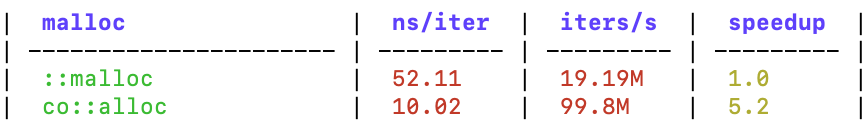Benchmark

include: co/benchmark.h.

## #basic concept

co.benchmark is a benchmark framework added in v3.0.1, which can be used for performance benchmarking.

### #BM_group

``````#define BM_group(_name_) \
......\
void _co_bm_group_##_name_(bm::xx::Group& _g_)
``````
• The `BM_group` macro is used to define a benchmark group, which actually defines a function.
• Multiple benchmarks can be defined with BM_add in each group.
The parameter `_name_` is the group name, which is also part of name of the defined function. `BM_group(atomic)` is ok, but `BM_group(co.atomic)` is not allowed, as `co.atomic` can’t be used in function name.

``````#define BM_add(_name_) \
_g_.bm = #_name_; \
``````
• The `BM_add` macro is used to define a benchmark, it must be used inside the function defined by BM_group.
The parameter `_name_` is the benchmark name, unlike `BM_group`, `BM_add(co.atomic)` is also allowed.

### #BM_use

``````#define BM_use(v) bm::xx::use(&v, sizeof(v))
``````
• The `BM_use` macro tells the compiler that the variable `v` will be used, which prevents the compiler from optimizing away some test code.

## #Write benchmark code

### #Code example

``````#include "co/benchmark.h"
#include "co/mem.h"

BM_group(malloc) {
void* p;

p = ::malloc(32);
);
BM_use(p);

p = co::alloc(32);
);
BM_use(p);
}

int main(int argc, char** argv) {
flag::parse(argc, argv);
bm::run_benchmarks();
return 0;
}
``````
• The code above defines a benchmark group called `malloc`, and 2 benchmarks are added to it with `BM_add`.
• Calling `bm::run_benchmarks()` will execute all the benchmark code.
In the above example, if there is no `BM_use(p)`, the compiler may think that `p` is an unused variable, and optimize away the relevant test code, resulting in the inability to measure accurate results.

### #Result example• The result of the benchmark test is printed as a markdown table, and it can be easily copied to a markdown document.
• Multiple `BM_group` will generate multiple markdown tables.
• The first column of the table is all benchmarks in the group, the second column is the time of a single iteration (in nanoseconds), the third column is the number of iterations per second, and the fourth column is the performance improvement multiple, based on the first benchmark.
•English